# 1) When two point charges are a distance d part, the electric force that each one feels from the other has magnitude F. In order to make this force twice as strong, the distance would have to be chan...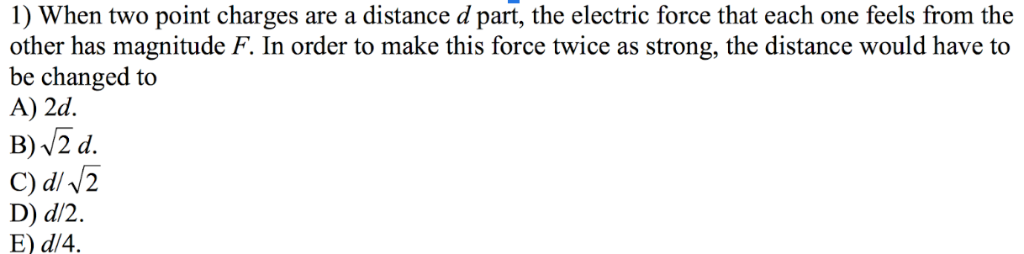1) When two point charges are a distance d part, the electric force that each one feels from the other has magnitude F. In order to make this force twice as strong, the distance would have to be changed to A) 2d. B) v2 d D) d/2. E) d/4.

Force between two charges is inversely proportional to square of distance between them

To make the force twice as strong, d/sqrt(2) should be the new distance. Option c is correct

##### Add Answer of: 1) When two point charges are a distance d part, the electric force that each one feels from the other has magnitude F. In order to make this force twice as strong, the distance would have to be chan...
Similar Homework Help Questions
• ### QUESTION 5 two point electric charges experience a force F when they are separated by a distance d. If this distance is increased to 3d, what will the force now be? F/9 3F Fr3 9F QUESTION 6 wo i...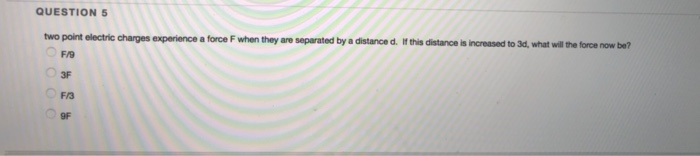QUESTION 5 two point electric charges experience a force F when they are separated by a distance d. If this distance is increased to 3d, what will the force now be? F/9 3F Fr3 9F QUESTION 6 wo identical charges experience a repuisive force of 0.29 N when separated by a distance of 59 om. What is the magnitude of the charges, In units of Coulombs7 QUESTION 5 two point electric charges experience a force F when they are separated...

• ### Two point charges are separated by a distance of 20.0 cm. The numerical value of one...

Two point charges are separated by a distance of 20.0 cm. The numerical value of one charge is twice that of the other. If each charge exerts a force of magnitude 45.0 N on the other, find the magnitude of the charges. please show diagram and each algebraic steps.

• ### Part A Constants Find the magnitude of the net electric field these charges produce at point...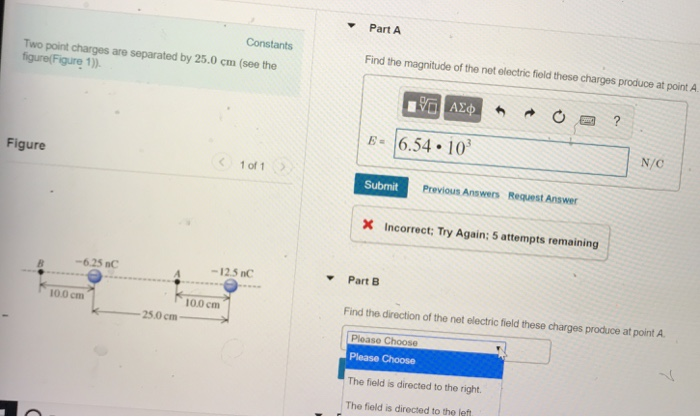Part A Constants Find the magnitude of the net electric field these charges produce at point A Two point charges are separated by 25.0 cm (see the figure(Figure 1)) 6.54. 10 N/C Figure 1 of 1 Submit Previous Answers Request Answer X Incorrect: Try Again; 5 attempts remaining -6.25 nC -12.5 nC Part B 10.0 cm 100 cm Find the direction of the net electric field these charges produce at point A 25.0cm Ploaso Please Choose The field is directed...

• ### Two charges, one twice as large as the other, are 15 cm apart, and each experiences...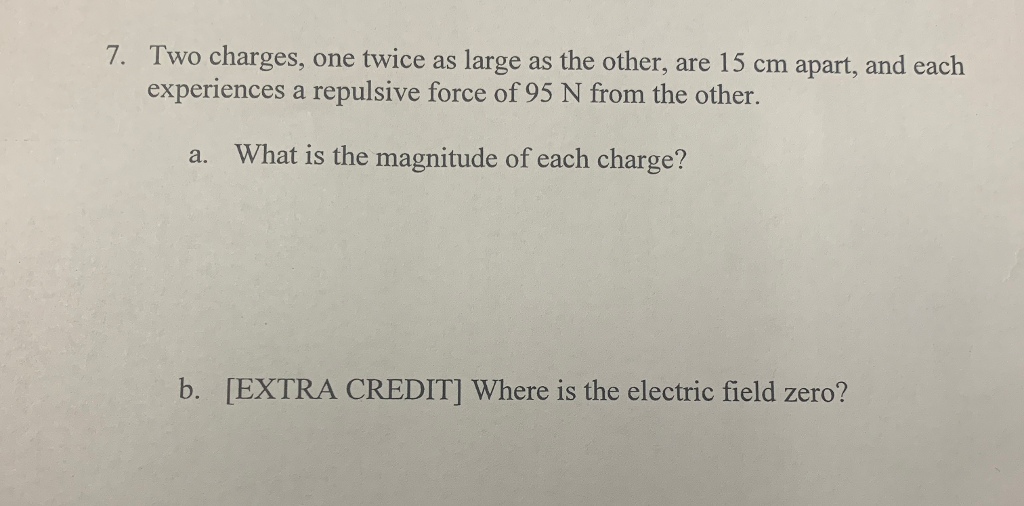Two charges, one twice as large as the other, are 15 cm apart, and each experiences a repulsive force of 95 N from the other. 7. What is the magnitude of each charge? a. b. [EXTRA CREDIT] Where is the electric field zero?

• ### 2. Calculate the electric force F. (magnitude and direction) between two point charges qi -10.0pC and...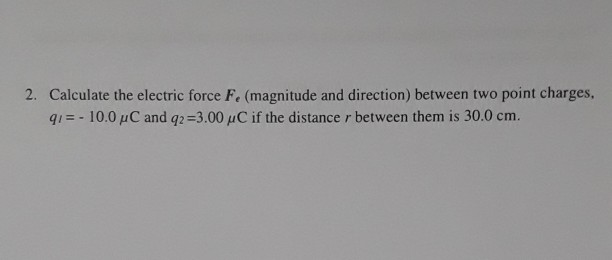2. Calculate the electric force F. (magnitude and direction) between two point charges qi -10.0pC and q,-3.00 C if the distance r between them is 30.0 cm.

• ### Two point charges are distance d = 59.3 cm apart, They have equal and opposite charge, each with...

Two point charges are distance d = 59.3 cm apart, They have equal and opposite charge, each with magnitude Q = 8.62 µC. The negative charge is on the left, and thepositive charge is on the right. An electron (mass 9.11x10-31 kg, charge 1.6x10-19 C) is placed half-way between the charges.a) The electric field at this point is (in N/C)b) The force on the electron is (in N)

• ### Two point charges are separated by a distance of 40.0 cm. The numerical value of one...

Two point charges are separated by a distance of 40.0 cm. The numerical value of one charge is twice that of the other. Each charge exerts a force of magnitude 65.0 N on the other. a.Find the magnitude of the charge with a smaller magnitude. (Express your answer to three significant figures.) b.Find the magnitude of the charge with a greater magnitude. (Express your answer to three significant figures.)

• ### Two point charges are separated by a distance of 40.0 cm. The numerical value of one...

Two point charges are separated by a distance of 40.0 cm. The numerical value of one charge is twice that of the other. Each charge exerts a force of magnitude 65.0 N on the other. a.Find the magnitude of the charge with a smaller magnitude. (Express your answer to three significant figures.) b.Find the magnitude of the charge with a greater magnitude. (Express your answer to three significant figures.)

• ### A pair of electrically charged objects attract each other with a force of 1 N when...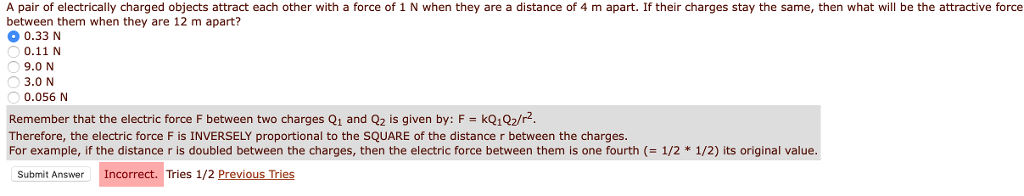A pair of electrically charged objects attract each other with a force of 1 N when they are a distance of 4 m apart. If their charges stay the same, then what will be the attractive force between them when they are 12 m apart? 0.33 N 0.11 N 9.0 N 3.0 N 0.056 N Remember that the electric force F between two charges Q1 and Q2 is given by: F kQ1Q2/r Therefore, the electric force F is INVERSELY proportional...

• ### Two point charges are separated by a distance of 50.0 cm. The numerical value of one...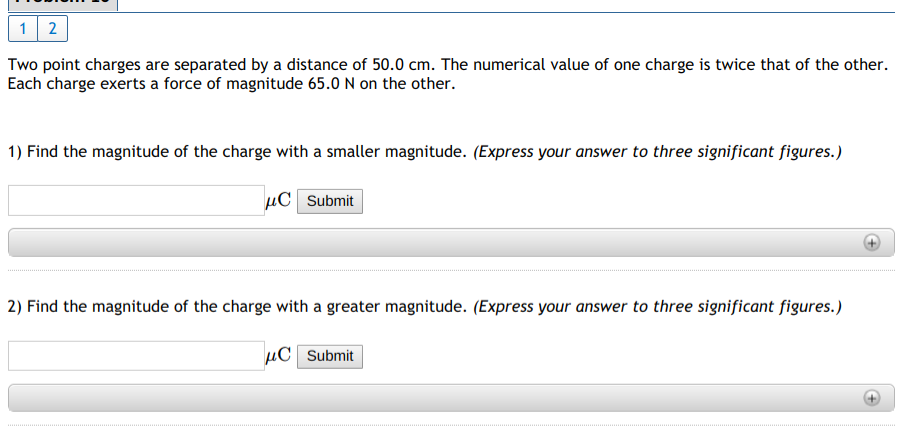Two point charges are separated by a distance of 50.0 cm. The numerical value of one charge is twice that of the other Each charge exerts a force of magnitude 65.0 N on the other. 1) Find the magnitude of the charge with a smaller magnitude. (Express your answer to three significant figures.) submit 2) Find the magnitude of the charge with a greater magnitude. (Express your answer to three significant figures.)

Need Online Homework Help?